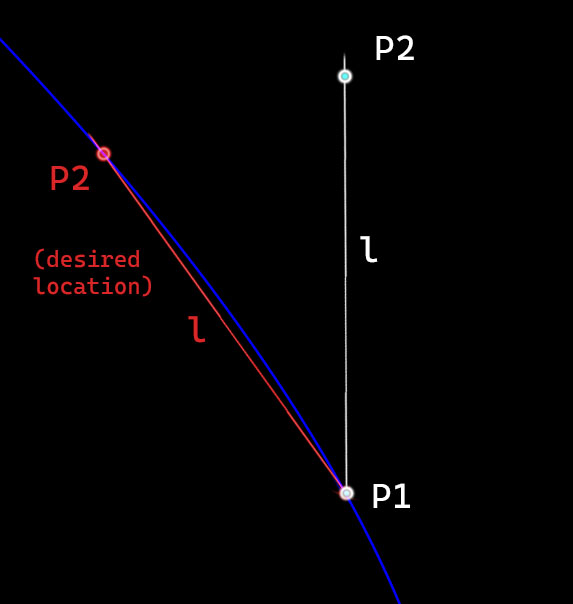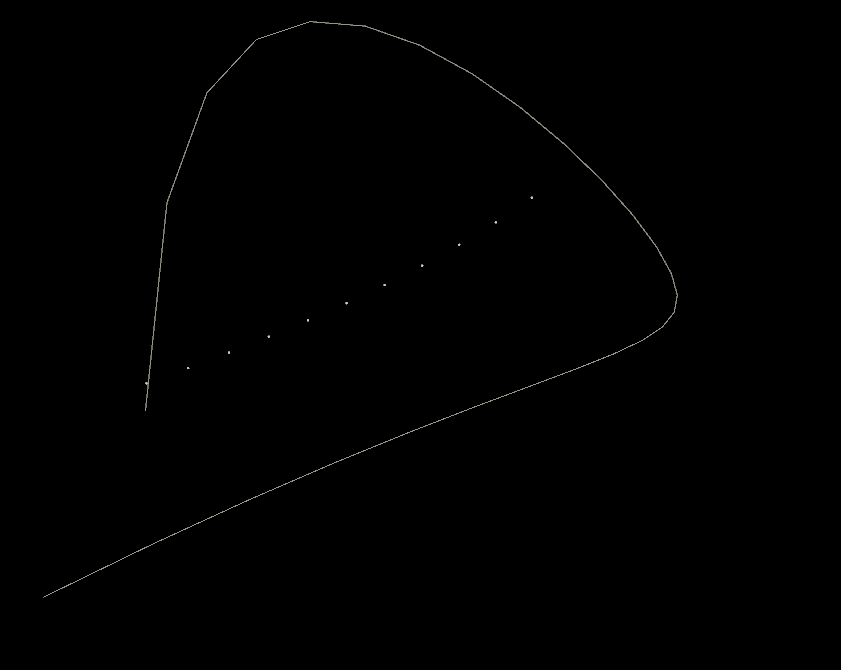# How to find the closest u-value on a curve intersecting a vector

## Recommended Posts

Hi

I'm trying to make a chain of points follow a curve, maintaining the distance between them.

I can achieve this in a rough way by using primuv/ primitive attribute in vops and offseting the U value by the distance. However this doesn't preserve the length L, due to the curvature.

How would I find the 'chord' points of the curve along the vector P1P2 ? Another way to think of this would be the intersection of the curve and a sphere of radius L.
Are there helper functions already part of Houdini that will allow me to do this?

Thanks##### Share on other sites

if you have a curve you can check the distance between p1 and the points of the curve.

if you resample your curve with lots of points you could select the points within some range from l, the more the points of your curve, the smaller the range you can use and the more precise it will be.

You could also find more than one point on the curve that is at distance "l", in that case you should select the one with the "u" parameter closer to whatever "u" value you have at p1.

You could also iteratively check if the difference between l and the distance form the world position at u value and p1 is within some range,  and progressively move along the u trying to reduce that difference, But i feel this could be slow because you have to call the function to read the u value for each iteration.

##### Share on other sites

Thanks for your reply. Do you know if I would I need to use nearpoints() for this and choose the last item of the array?

Or is there a more efficient function to use? Everything seems to allow for a maximum search distance but not a minimum.

##### Share on other sites

Hi,

you can try to iterate. Start with a first try and find the u-value, where the length between the u-values matches the length of the vector.

Here is some VEX Code. Peri is the length of the curve (you can use measure here). L is the length of the vector. u the position of P1. This will surely not work in every situation. But if you start with L / Peri, you have a first guess and probably length between the curve points is greater. So the next du should be a bit bigger. If the length are same, the du should not change anymore.

```float get_deltau(float u, L, Peri)
{
float du = L / Peri;
int maxcount = 10;
int count = 0;
while (count < maxcount)
{
vector A = primuv(1, 'P', 0, u);
vector B = primuv(1, 'P', 0, u + du);
float delta_u = L / length(A - B) * du;
du = delta_u;
count += 1;
}
return du;

}```

P.S. it will be better to add a stop condition in the function and also apply resample on the curve.

If interested here is a modification of an older experimental file of my collection.

objects_along_curveC.hipnc

Edited by Aizatulin
•1

##### Share on other sites

Thanks, I'll give it a go and report backWas reluctant to do an iterative approach but might be the only way!

##### Share on other sites

here’s another one without relying on an iterative approach

•5

##### Share on other sites

@petz: Thanks for the insight.
Purely out of curiosity: Would it be beneficial to separate generating matrices and actual point transformations?

```// calculate transform matrices per class in DETAIL wrangle
float offset = chf('offset');

4[]@xform = {};

int num = nuniqueval(0, 'prim', 'class');
float dist_sum = offset;
for(int i = 0; i < num; i++){
int class = uniqueval(0, 'prim', 'class', i);
string grp = sprintf("@class==%g", class);
float size = vector(getbbox_size(0, grp)).z;
dist_sum += size * 0.5;
float u = primuvconvert(1, dist_sum, 0, 10);
vector pos = primuv(1, 'P', 0, u);
vector tangent = normalize(primuv(1, 'tangentu', 0, u));
matrix m = matrix(dihedral({0,0,1}, tangent));
translate(m, pos);
append(@xform, m);
dist_sum += size * 0.5;
}```
```// apply transforms in POINT wrangle
matrix xforms[] = detail(0, 'xform', 0);
int class = prim(0, 'class', i@primnum);

v@P *= xforms[class];```precalculate_transforms.hipnc

##### Share on other sites
17 hours ago, konstantin magnus said:

Purely out of curiosity: Would it be beneficial to separate generating matrices and actual point transformations?

with high resolution geometry most probably yes, in the example i’ve posted above i guess not.
however, if speed is important it might be best to skip the second wrangle at all and use copyToPoints instead. packing the geo beforehand might also give you some speed improvements (in both cases) ...

Edited by petz
•1

##### Share on other sites

@petz this is great thanks - didn't know about primuvconvert##### Share on other sites

Hi @Petz,

nice approach but I had no success to make it work the way as I understood the question.
My goal was to to create a chain on a curve, where the start and the end point of each object should stay on the curve and the distance between the points should
stay the same. I know that this is not possible for every case, but it will be interesting, if this will work without iteration.
The result should be equivalent (as described) to intersections with a sphere around the starting point.
The problem is how to find the right point. My aproach was to start with a guess (length of curve divided by the object length, where the difference between those point should be always smaller than the partial curve length).
Get the ratio between the the object length and the distance between the points. If the ratio higher, the u-value is too small and vice versa. But this approach may not converge properly.
Bisection method can be added aswell (once both sides have been detected), which always converges but it returns any solution.

To visualize -> here is another example using straight lines

objects_along_curve_connected_start_end_maintain_length.hipnc

##### Share on other sites

Yeah that was my intended use case too, with points. It seems like I'm almost there but getting a bit confused. What is primduv used for, aka 'position derivative'?

```float dist = ch("dist");
for(int i = 0; i < @numpt; i++) //removed group code and simply run this over the input points
{

float point_dist = point(0,"distance",i) // added interpoint distance as attribute;
vector size = set(point_dist,0,0);

dist += point_dist;

float u_unit = primuvconvert(1, dist, 0, 10); //changed to the 1D form of primuvconvert

vector pos = primuv(1, "P", 0, u_unit);

vector tangent = normalize(primduv(@OpInput2, 0, u_unit, 1, 0));

matrix mat = matrix(dihedral({1, 0, 0}, tangent)); //changed vector-a to x direction (input points go that way)
translate(mat, pos);

pos = point(0, "P", i);
pos *= mat;
setpointattrib(0, "P", i, pos, "set");

}```

Looks like it's rotated from where it should be:Using Petz' code, a workaround could be converting the input points to spheres with radius `@joint_length`  , then replacing the transformed spheres with their center points. But I imagine at that point it's quicker to use the iterative way?

geo_along_curve_edited.hipnc

Edited by danvenn

##### Share on other sites

Here are some issues. In dihedral you are using x-aligned, but your line is z-aligned. The other thing is, that the points you are applying the matrix on, are getting more away from the center, when the point number increases, so you probably want to subtract the predecessor point(i-1) (if exists), before applying the rotation.

ps: and yes primduv is getting the derivative (~tangent)

Edited by Aizatulin

##### Share on other sites

Thanks for the advice. Taken a break this week but this is today's progress. The points behave except for pt, and there's an offset starting from pt.

I simplified to the code down to this:

```float offset = ch("dist");
for(int i = 0; i < @numpt; i++)
{

float point_dist = point(0,"cumuldist",i); //

float totaldist = offset + point_dist;

//get U on curve using arclength
float u_unit = primuvconvert(1, totaldist, 0, 10); //

//get position of U on curve
vector pos = primuv(1, "P", 0, u_unit);

//get tangent vector on curve at U.
vector tangent = normalize(primduv(@OpInput2, 0, u_unit, 1, 0));

setpointattrib(0, "P", i, pos, "set");

}```

This works quite well but is a bit inaccurate because it uses the u-value taken from the arclength. I added a cumulative distance attribute beforehand. I might rewrite the rotation section I took out to a method I understand a bit better.

##### Share on other sites

You don't need the rotation at all, if you are mapping the points directly on the curve. But keep in mind, that the distance between two points is usually different (smaller) compared to the length of the curve section between both points.

##### Share on other sites
On 26.1.2021 at 4:30 PM, Aizatulin said:

Hi @Petz,

nice approach but I had no success to make it work the way as I understood the question.
My goal was to to create a chain on a curve, where the start and the end point of each object should stay on the curve and the distance between the points should
stay the same. I know that this is not possible for every case, but it will be interesting, if this will work without iteration.
The result should be equivalent (as described) to intersections with a sphere around the starting point.
The problem is how to find the right point. My aproach was to start with a guess (length of curve divided by the object length, where the difference between those point should be always smaller than the partial curve length).

well, in this case there is no smart analytical method i know about. what you could try to do is to interpolate a polynomial and solve for the given “chord” length. but i doubt it would be any faster than iteratively searching for the distance …

•1

## Create an account

Register a new account